## 纪念年初陨落的计量经济学大师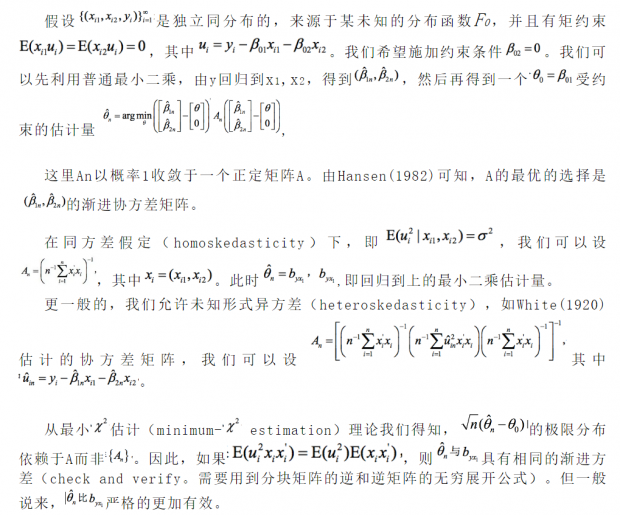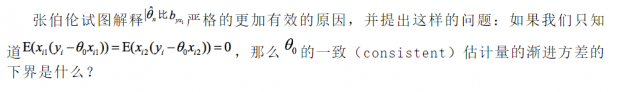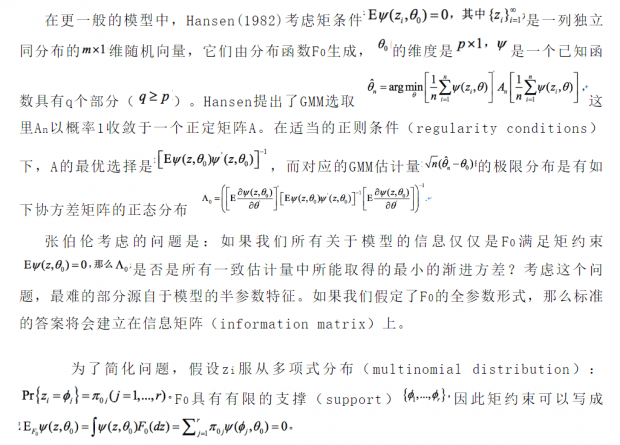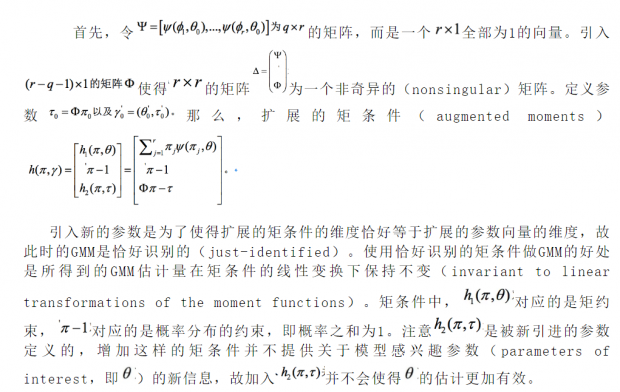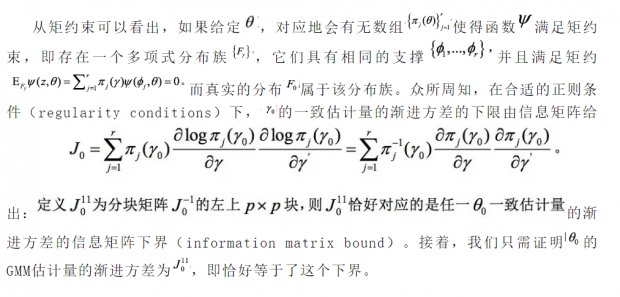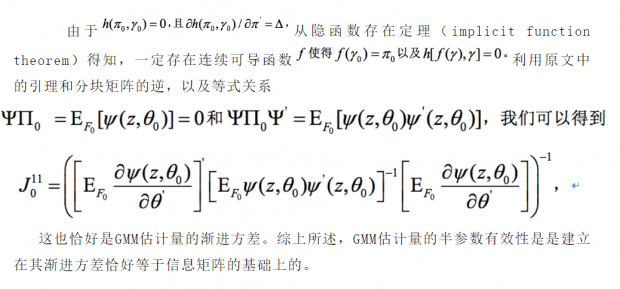Abstract

In this paper, bounds on asymptotic efficiency are derived for a class of non-parametric models. The data are independent and identically distributed according to some unknown distribution F. There is a given function of the data and a parameter. The restrictions are that a conditional expectation of this function is zero at some point in the parameter space; this point is to be estimated. If F is assumed to be a multinomial distribution with known (finite) support, then the problem becomes parametric and the bound can be obtained from the information matrix. This bound turns out to depend only upon certain conditional moments, and not upon the support of the distribution. Since a general F can be approximated by a multinomial distribution, the multinomial bound applies to the general case.